# Selina Concise Mathematics Class 6 ICSE Solutions Chapter 27 Quadrilateral

## Selina Concise Mathematics Class 6 ICSE Solutions Chapter 27 Quadrilateral

Selina Publishers Concise Mathematics Class 6 ICSE Solutions Chapter 27 Quadrilateral

### Quadrilateral Exercise 27A – Selina Concise Mathematics Class 6 ICSE Solutions

Question 1.
Two angles of a quadrilateral are 89° and 113°. If the other two angles are equal; find the equal angles.
Solution: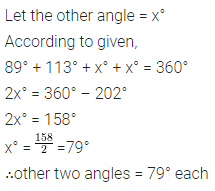Question 2.
Two angles of a quadrilateral are 68° and 76°. If the other two angles are in the ratio 5 : 7; find the measure of each of them.
Solution:Question 3.
Angles of a quadrilateral are (4x)°, 5(x+2)°, (7x-20)° and 6(x+3)°. Find
(i) the value of x.
(ii) each angle of the quadrilateral.
Solution:Question 4.
Use the information given in the following figure to find :
(i) x
(ii) ∠B and ∠C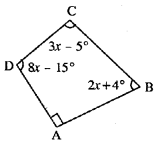Solution:Question 5.
In quadrilateral ABCD, side AB is parallel to side DC. If ∠A : ∠D = 1 : 2 and ∠C : ∠B = 4:5
(i) Calculate each angle of the quadrilateral.
(ii) Assign a special name to quadrilateral ABCD.
Solution:Question 6.
From the following figure find ;
(i) x,
(ii) ∠ABC,
(iii) ∠ACD.
Solution: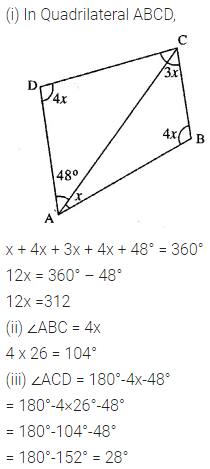Question 7.
Given : In quadrilateral ABCD ; ∠C = 64°, ∠D = ∠C – 8° ;
∠A = 5(a+2)° and ∠B = 2(2a+7)°.
Calculate ∠A.
Solution:
</span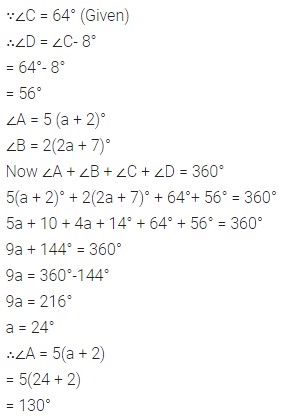Question 8.
In the given figure :
∠b = 2a + 15
and ∠c = 3a+5; find the values of b and c.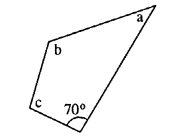Solution: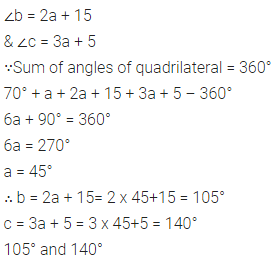Question 9.
Three angles of a quadrilateral are equal. If the fourth angle is 69°; find the measure of equal angles.
Solution:Question 10.
In quadrilateral PQRS, ∠P : ∠Q : ∠R : ∠S = 3 : 4 : 6 : 7.
Calculate each angle of the quadrilateral and then prove that PQ and SR are parallel to each other. Is PS also parallel to QR ?
Solution:Question 11.
Use the information given in the following figure to find the value of x.
Solution:Question 12.
The following figure shows a quadrilateral in which sides AB and DC are parallel.
If ∠A : ∠D = 4 : 5, ∠B = (3x – 15)° and ∠C = (4x + 20)°, find each angle of the quadrilateral ABCD.Solution:### Quadrilateral Exercise 27B – Selina Concise Mathematics Class 6 ICSE Solutions

Question 1.
In a trapezium ABCD, side AB is parallel to side DC. If ∠A = 78° and ∠C = 120°, find angles B and D.
Solution:Question 2.
In a trapezium ABCD, side AB is parallel to side DC. If ∠A = x° and ∠D = (3x – 20)°; find the value of x.
Solution:Question 3.
The angles A, B, C and D of a trapezium ABCD are in the ratio 3 : 4 : 5 : 6.
Le. ∠A : ∠B : ∠C : ∠D = 3:4: 5 : 6. Find all the angles of the trapezium. Also, name the two sides of this trapezium which are parallel to each other. Give reason for your answer
Solution: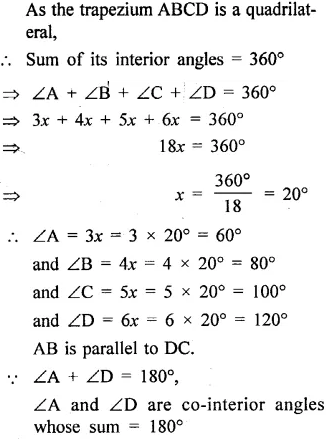Question 4.
In an isosceles trapezium one pair of opposite sides are ….. to each Other and the other pair of opposite sides are ….. to each other.
Solution: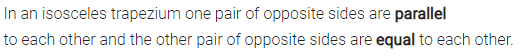Question 5.
Two diagonals of an isosceles trapezium are x cm and (3x – 8) cm. Find the value of x.
Solution: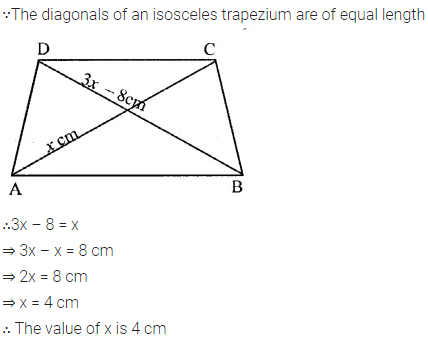Question 6.
Angle A of an isosceles trapezium is 115° ; find the angles B, C and D.
Solution: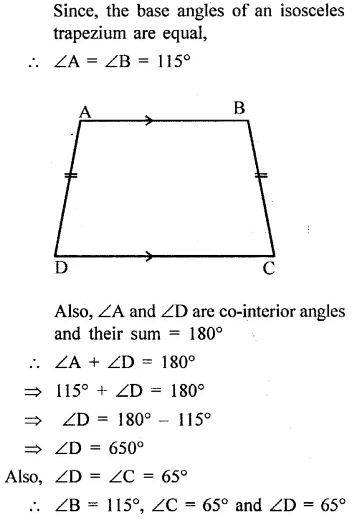Question 7.
Two opposite angles of a parallelogram are 100° each. Find each of the other two opposite angles.
Solution:Question 8.
Two adjacent angles of a parallelogram are 70° and 110° respectively. Find the other two angles of it.
Solution: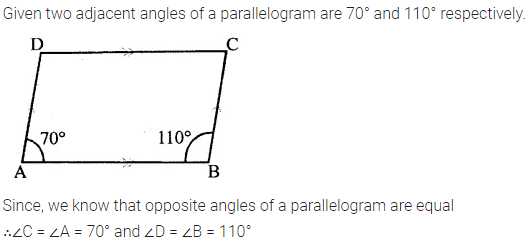Question 9.
The angles A, B, C and D of a quadrilateral are in the ratio 2:3: 2 : 3. Show this quadrilateral is a parallelogram.
Solution: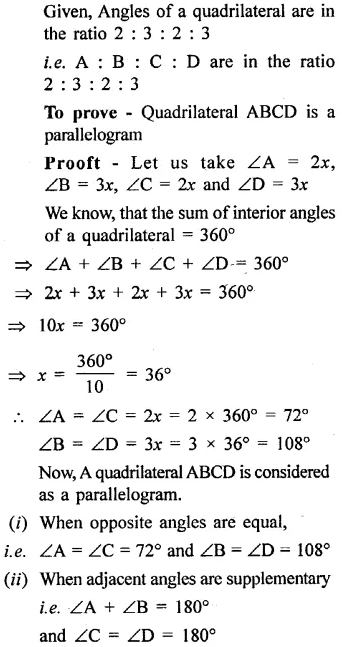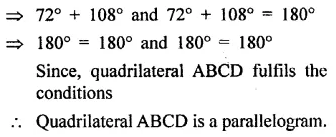Question 10.
In a parallelogram ABCD, its diagonals AC and BD intersect each other at point O.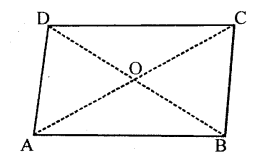If AC = 12 cm and BD = 9 cm ; find; lengths of OA and OD.
Solution:Question 11.
In parallelogram ABCD, its diagonals intersect at point O. If OA = 6 cm and OB = 7.5 cm, find the length of AC and BD.Solution: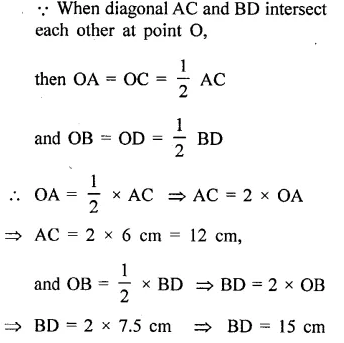Question 12.
In parallelogram ABCD, ∠A = 90°
(i) What is the measure of angle B.
(ii) Write the special name of the paralleogram.
Solution: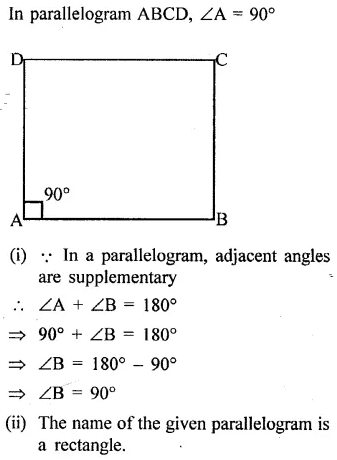Question 13.
One diagnol of a rectangle is 18 cm. What is the length of its other diagnol?
Solution:Question 14.
Each angle of a quadrilateral is x + 5°. Find :
(i) the value of x
(ii) each angle of the quadrilateral.
Give the special name of the quadrilateral taken.Solution: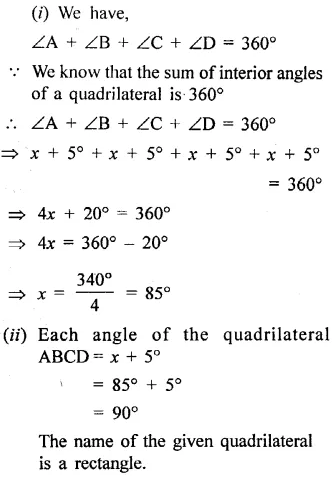Question 15.
If three angles of a quadrilateral are 90° each, show that the given quadrilateral is a rectangle.
Solution: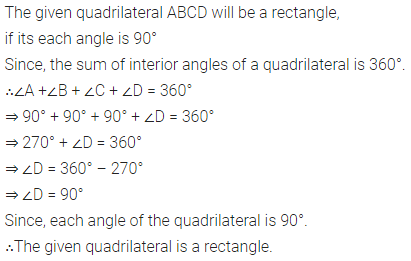Question 16.
The diagnols of a rhombus are 6 .cm and 8 cm. State the angle at which these diagnols intersect.
Solution:Question 17.
Write, giving reason, the name of the figure drawn alongside. Under what condition will this figure be a square.Solution:Question 18.
Write two conditions that will make the adjoining figure a square.Solution: